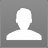Categoty : Algebra
elaine : the no of ordered pairs (m,n) where m,n belongs to {1,2,3,.....,100} such that 7^m + 7^n is divisible b by 5 is ?: the no of ordered pairs (m,n) where m,n belongs to {1,2,3,.....,100} such that 7^m + 7^n is divisible b by 5 is ? Let ( 3, 2) be (x subscript 1 space comma space y subscript 1 space) and ( -1, 4) be left parenthesis x subscript 2 comma space y space subscript 2 right parenthesis. Then, the equation of the line passing through ( x subscript 1 comma space space space end subscript y subscript 1 space end subscript) and (x subscript 2 comma space y subscript 2) is fraction numerator y space minus space y subscript 1 over denominator y subscript 1 space minus end subscript space y subscript 2 end fraction space equals space fraction numerator x space minus x subscript 1 over denominator x subscript 1 minus space x subscript 2 end fraction Substituting the values of x subscript 1 comma space y subscript 1 comma space y subscript 2 comma space y subscript 2 comma end subscript we get, fraction numerator y space minus space left parenthesis negative 2 right parenthesis over denominator negative 2 minus 4 end fraction space equals space fraction numerator x space minus space 3 over denominator 3 minus left parenthesis negative 1 right parenthesis end fraction fraction numerator y space plus space 2 over denominator negative 6 space end fraction space equals space fraction numerator x space minus 3 over denominator 4 end fraction rightwards double arrow 4( y+2) = -6(x-3) rightwards double arrow4y +8 = -6x +18 rightwards double arrow2y +3x = 5 Ans